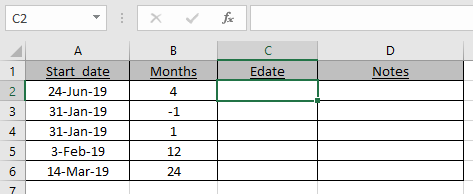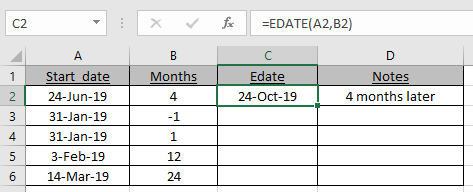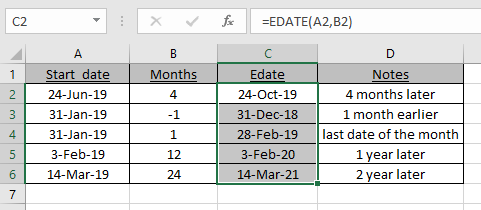# How to use the EDATE function in Excel

EDATE function returns the date after adding or subtracting months on a given date.

The EDATE function takes the date as an argument and edits n months to the date.
Syntax:

=EDATE (date, months)

Date : date_value
Months : months to edit
Let’s understand this function using it in an example.Here we have some dates and we need to find the Edate value for the given dates and months.
Use the formula:

=EDATE(A2,B2)

A2 : Given date
B2 : number of monthsAs you can see the new_date or EDATE is here.

Now use the formula to the remaining cells to get the EDATE for a given date.As you can see in C4 cell, 31st Jan becomes 28th Feb as 28th is the last date of the Feb.

EDATE formula gets you the date of expiry, maturity dates or other due dates.

I hope we are clear on how to use the MIRR function and in Excel. Explore more articles on Excel financial functions here. Please feel free to state your query or feedback for the above article.

Related Articles:

Calculate Months Between dates in Excel

Get days between dates in Excel

How to use the DAYS function in Excel

Popular Articles:

How to use the VLOOKUP Function in Excel

How to use the COUNTIF function in Excel 2016

How to Use SUMIF Function in Excel

Terms and Conditions of use

The applications/code on this site are distributed as is and without warranties or liability. In no event shall the owner of the copyrights, or the authors of the applications/code be liable for any loss of profit, any problems or any damage resulting from the use or evaluation of the applications/code.